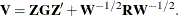### WEIGHT Statement

`WEIGHT` variable ;

If you do not specify a REPEATED statement, the WEIGHT statement operates exactly like the one in PROC GLM. In this case PROC MIXED replacesand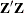with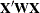and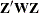, where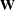is the diagonal weight matrix. If you specify a REPEATED statement, then the WEIGHT statement replaceswith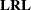, whereis a diagonal matrix with elements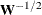. Observations with nonpositive or missing weights are not included in the PROC MIXED analysis.

If a computation in PROC MIXED involves, then the WEIGHT statement replaceswith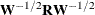. For example, the covariance matrix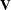for the observations usually have the form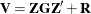, which with the WEIGHT statement becomes